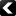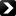Under the auspices of the Computational Complexity Foundation (CCF)LATEST > REPORTS:PreviousNextTR21-140 | 27th September 2021
Nathan Geier

#### Tight Computational Indistinguishability Bound of Product Distributions

Assume that $X_0,X_1$ (respectively $Y_0,Y_1$) are $d_X$ (respectively $d_Y$) indistinguishable for circuits of a given size. It is well known that the product distributions $X_0Y_0,\,X_1Y_1$ are $d_X+d_Y$ indistinguishable for slightly smaller circuits. However, in probability theory where unbounded adversaries are considered through statistical distance, it is folklore knowledge that in ... more >>>

TR21-139 | 24th September 2021
Venkatesan Guruswami, Jonathan Mosheiff

#### Punctured Large Distance Codes, and Many Reed-Solomon Codes, Achieve List-Decoding Capacity

We prove the existence of Reed-Solomon codes of any desired rate $R \in (0,1)$ that are combinatorially list-decodable up to a radius approaching $1-R$, which is the information-theoretic limit. This is established by starting with the full-length $[q,k]_q$ Reed-Solomon code over a field $\mathbb{F}_q$ that is polynomially larger than the ... more >>>

TR21-138 | 23rd September 2021
Rahul Santhanam, Iddo Tzameret

#### Iterated Lower Bound Formulas: A Diagonalization-Based Approach to Proof Complexity

We propose a diagonalization-based approach to several important questions in proof complexity. We illustrate this approach in the context of the algebraic proof system IPS and in the context of propositional proof systems more generally.

We give an explicit sequence of CNF formulas $\{\phi_n\}$ such that VNP$\neq$VP iff there are ... more >>>PreviousNextISSN 1433-8092 | Imprint